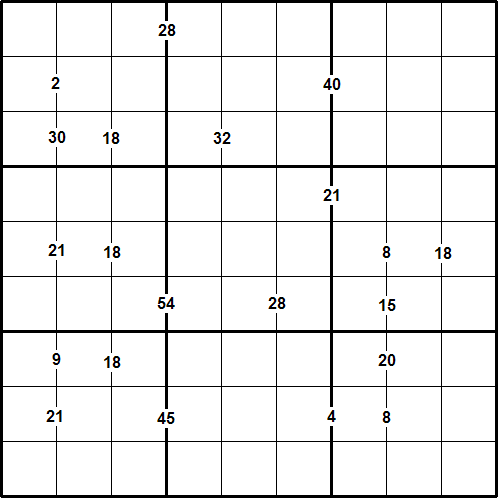#### You may also like### Repeaters

Choose any 3 digits and make a 6 digit number by repeating the 3 digits in the same order (e.g. 594594). Explain why whatever digits you choose the number will always be divisible by 7, 11 and 13.### Oh! Hidden Inside?

Find the number which has 8 divisors, such that the product of the divisors is 331776.### Skeleton

Amazing as it may seem the three fives remaining in the following `skeleton' are sufficient to reconstruct the entire long division sum.

# Multiples Sudoku

##### Age 11 to 14 Challenge Level:

Discovered by Henry Kwok#### Rules of Multiples Sudoku

Like a conventional Sudoku, this Multiples Sudoku has two basic rules:
1. Each column, row, and 3 x 3 subgrid must have the numbers 1 to 9.
2. No column, row, or subgrid can have two cells with the same number.

The puzzle can be solved with the help of  the numbers which are placed on the border lines between selected pairs of neighbouring cells.
These numbers are the product of the two digits in the cells to the left and right of the clue.

For example, where there is a 12 on the line between two neighbouring cells, the cells must contain 2 and 6, or 6 and 2, or 3 and 4, or 4 and 3.

After finding the values of all the unknown digits, the puzzle is solved by the usual Sudoku strategy.

A printable version of the problem can be found here.